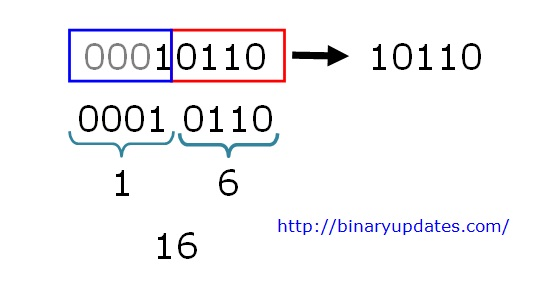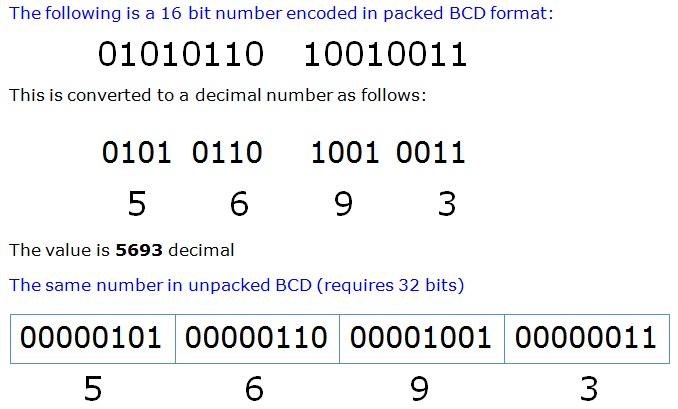Friday , May 24 2019

# Number System in Embedded Programming

It’s important for everyone to make sure that they have understood Number Systems and Computer Data Representation. Here we’ll learn everything about different types of Number System in Embedded Programming. There are different ways you can represent computer data say for example: Binary, Decimal, Hexadecimal and BCD (Binary Coded Decimal) Numbers etc. We’ll explore each of them with their inter-conversion.

#### Decimal Number System

Decimal numbering system uses digits from 0 to 9 i.e. (0, 1, 2, 3, 4, 5, 6, 7, 8, and 9). The base of decimal number system is 10 because we use 10 digits to represent decimal number. When we write decimal numbers, we use a positional notation system. Each digit is multiplied by an appropriate power of 10 depending on its position in the number. Let’s take example: 5319

 5319 = 5 x 103 + 3 x 102 + 1 x 101 + 9 x 100 = 5 x 1000 + 3 x 100 + 1 x 10 + 9 x 1 = 5000 + 300 + 10 + 9

For whole number, the rightmost digit is 1st position (100 = 1). The numeral in that position indicates how many ones are present in the number. The next position to the left is ten’s, then hundred’s, thousand’s, and goes on. Each digit position has a weight that is ten times the weight of the position to its right.

In the decimal number system, there are ten possible values that can appear in each digit position, and so there are ten numerals required to represent the quantity in each digit position. The decimal numerals are the familiar zero through nine (0, 1, 2, 3, 4, 5, 6, 7, 8 and 9).

#### Binary Number System

Binary Numbers uses only 0 and 1 digit. The base of binary number system is 2. The binary number system is also a positional notation numbering system. Each digit position in a binary number represents a power of two. So, when we write a binary number, each binary digit is multiplied by an appropriate power of 2 based on the position in the number. Example: Find decimal equivalent of binary number 10101

 10101 = 1 x 24 + 0 x 23 + 1 x 22 + 0 x 21 + 1 x 20 = 1 x 16 + 0 x 8 + 1 x 4 + 0 x 2 + 1 x 1 = 16 + 4 + 1

The decimal equivalent of 10101 is 21. Each digit in binary number carries specific multiplication factor. By default the bit on extreme right which is also called as LSB bit or 0th bit and that on the extreme left is MSB or last bit. SO technically in computing first bit will be 0th index and hence the right will have an order of 20, next bit will have an order of 21 and 22, 23 and so on. And when we start conversion we have to consider from LSB i.e. from RIGHT we multiply each bit with increasing power of 2 so Bit 0 will be multiplied with 20, Bit 1 will be multiplied with 21 and so on.

#### Hexadecimal Number System

Hexadecimal Number System uses 16 digits from 0 to 9 and A to F. The alphabets A to F represent decimal numbers from 10 to 15. The base of Hexadecimal uNmber system is 16. There are sixteen numerals required. Here are the hexadecimal numerals: 0, 1, 2, 3, 4, 5, 6, 7, 8, 9, A, B, C, D, E, F.

The reason for the common use of hexadecimal numbers is the relationship between the numbers 2 and 16. Sixteen is a power of 2 (16 = 24). Because of this relationship, four digits in a binary number can be represented with a single hexadecimal digit. This makes conversion between binary and hexadecimal numbers very easy, and hexadecimal can be used to write large binary numbers with much fewer digits. When working with large digital systems, such as computers, it is common to find binary numbers with 8, 16 and even 32 digits. Writing a 16 or 32 bit binary number would be quite tedious and error prone. By using hexadecimal, the numbers can be written with fewer digits and much less likelihood of error. This makes programmer life easy while writing programs.

##### Binary to Hexadecimal Numbers

Example: Find Hexadecimal equivalent of 10110, where 10110 is binary number.Binary to Hexadecimal Example

To convert any binary number into its equivalent hexadecimal number, we first need to group the bits each group will have 4-bits. In our example we have 5 binary digits, so we will group them into 00010110. After grouping bits directly replace group with its equivalent hexadecimal number. Make sure we always start grouping from RIGHT i.e. LSB bit to LEFT i.e. MSB bit. Hence the hex equivalent of 10110 is 16 or (0x16).

In the C and C++ languages, hexadecimal constants are represented with a ‘0x’ preceding the number, as in: 0x16.

Equivalent Numbers in Decimal, Binary, Hexadecimal Notations,

 Decimal Binary Hexadecimal 0 00000000 00 1 00000001 01 2 00000010 02 3 00000011 03 4 00000100 04 5 00000101 05 6 00000110 06 7 00000111 07 8 00001000 08 9 00001001 09 10 00001010 0A 11 00001011 0B 12 00001100 0C 13 00001101 0D 14 00001110 0E 15 00001111 0F 16 00010000 10 17 00010001 11 31 00011111 1F 32 00100000 20 63 00111111 3F 64 01000000 40 65 01000001 41 127 01111111 7F 128 10000000 80 129 10000001 81 255 11111111 FF 256 0000000100000000 0100 32767 0111111111111111 7FFF 32768 1000000000000000 8000 65535 1111111111111111 FFFF

#### BCD- Binary Coded Decimal Number System

BCD is Binary Coded Decimal. In BCD system, numbers are represented in decimal form. However, each decimal digit is encoded using a 4-bit binary number. Here is an example. The decimal number 568 would be represented in BCD as follows:

 568 = 0101  0110 1000 =     5        6        8

Conversion of numbers between decimal and BCD is quite simple. To convert from decimal to BCD, simply write down the 4-bit binary pattern for each decimal digit. To convert from BCD to decimal, divide the number into groups of 4 bits and write down the corresponding decimal digit for each 4-bit group.

There are a couple of variations on the BCD representation, namely packed and unpacked. An unpacked BCD number has only a single decimal digit stored in each data byte. In this case, the decimal digit will be in the low four bits and the upper 4-bits of the byte will be 0. In the packed BCD representation, two decimal digits are placed in each byte. Generally, the high order bits of the data byte contain the more significant decimal digit.BCD Number System

BCD numbers is not as common as binary number system because it is not space efficient. In packed BCD format, only 10 of the 16 possible bit patterns in each 4-bit unit are used. In unpacked BCD, only 10 of the 256 possible bit patterns in each byte are used. A 16-bit quantity can represent the range 0-65535 in binary, 0-9999 in packed BCD and only 0-99 in unpacked BCD.

This is it for Number system in Embedded Programming. Now we hope you’ve understood the concept of Binary, BCD and Hexadecimal Number Systems with its inter-conversion. Please do write us if you have any suggestion/comments or come across any error on this page.

### About Umesh LokhandeUmesh Lokhande holds a Master degree in Scientific Instrumentation from University of Applied Sciences Jena, Germany. and has previously worked at Orbotech, Alere Technologies etc. Umesh is also a founder and first author of BINARYUPDATES.COM